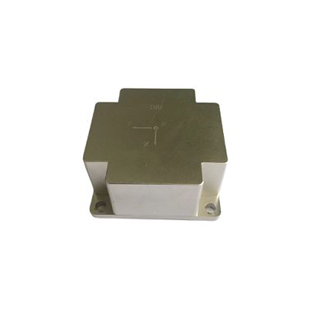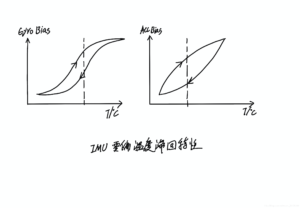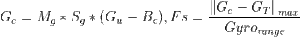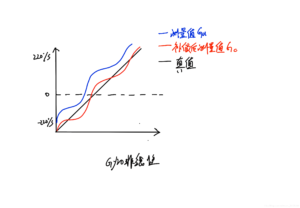Application

# How to choose MEMS IMUIMU (Inertial Measurement Unit) is an inertial measurement unit, which can measure the three-axis acceleration and angular velocity of an object. It is generally used in the measurement link of the system to estimate the pose of the object. The IMU generally includes a three-axis accelerometer and a three-axis gyroscope. The accelerometer detects the acceleration signal of the object in the independent three-axis of the carrier coordinate system, and the gyroscope detects the angular velocity signal of the carrier relative to the navigation coordinate system. The angular velocity and acceleration in space can solve the pose of the object. MEMS IMUs are cheap and small in size, and are widely used in many fields such as navigation, drones, VR, robots, and smart bracelets. The detection accuracy of the IMU is very important to the overall performance of the system. If the noise detected by the IMU is very noisy, the feedback the system gets is wrong, just like the human eyes, ears and other senses get the wrong information. How can we act freely? The bottom layer of the system is the foundation. If the bottom layer of the system is unstable, it will be difficult for the upper layer to perform well. ERICCO has always strictly controlled the accuracy of IMU, and has been pursuing to improve the system of IMU. Next, ERICCO will also launch new high-precision IMU products.

1.Zero bias temperature hysteresis characteristics

The zero-bias temperature hysteresis characteristic refers to the zero-bias inconsistency of the IMU corresponding to the temperature-rising phase and the temperature-falling phase. As shown in the figure, the horizontal axis is temperature, and the vertical axis is zero offset. Some IMU data sheets will give the zero-bias temperature hysteresis characteristic curve, some will not give it, it is best to test it when applying the IMU. Because the estimation of the IMU zero bias is calibrated according to the temperature (the IMU calibration algorithm is described in detail), if the temperature hysteresis difference is not too large, the calibration accuracy will be relatively high; if the IMU zero bias hysteresis value is too large , the IMU zero calibration error will be relatively large, thus affecting the effect of fusion.2.Vibration characteristics

In the case of external vibration, the variation characteristics of IMU bias with vibration frequency. Some MEMS IMU chips have abnormal frequency characteristics under high frequency excitation. For applications where high frequency vibration is prone to occur, such as rotor drones, the vibration characteristics are generally tested. If the IMU has abnormal frequency characteristics, it can be considered. Add shock absorber.

3.The effect of repeated power-on on IMU bias

Ideally, it is considered that under the same external conditions, the bias of the IMU will remain the same each time it is powered on. In practice, the bias of the IMU will be different each time the IMU is powered on under the same external conditions. If the difference is relatively large, it will be zero. The bias estimation error will be relatively large, which will affect the fusion effect.

4.The effect of stress on bias

The influence of stress on the IMU includes: the influence of the stress moment on the bias, and the influence of different stresses on the bias. The stress mainly comes from: the stress exerted by the PCB board on the IMU chip, and the stress exerted by the temperature control device on the IMU chip. If the IMU bias is too sensitive to the influence of stress, it will also affect the zero drift estimation error, thereby affecting the fusion effect.

5.Impact of impact on zero offset

When the IMU receives an external shock (on the order of dozens of G), there will be a probability that the IMU will be stuck, or the bias will change. Generally, it should be tested.

6.Nonlinear factor (%Fs)

Ideally, we think that the sensor data is linear within the range. In practice, the change of the sensor is nonlinear. As shown in Figure 2, it is necessary to test its nonlinear characteristics before using the IMU. If the nonlinearity is too serious, it should be For nonlinear calibration, there are many such calibration methods, such as proportional calibration, quadratic fitting calibration, etc. The following is an example of the simplest proportional calibration: